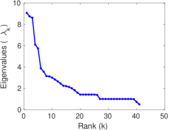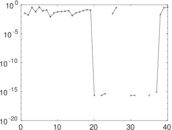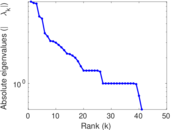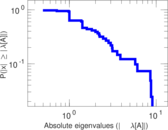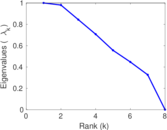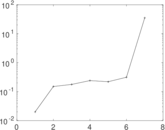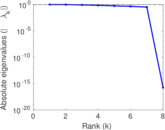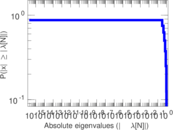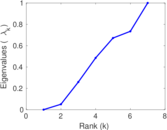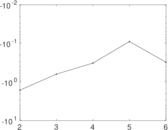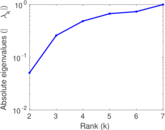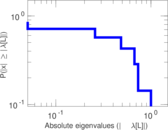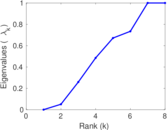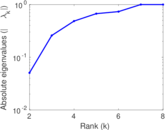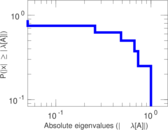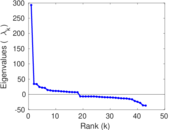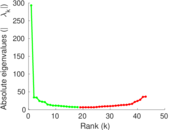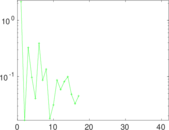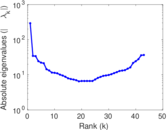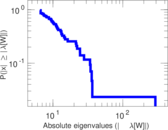# Wiktionary edits (as)

This is the bipartite edit network of the Assamese Wiktionary. It contains users and pages from the Assamese Wiktionary, connected by edit events. Each edge represents an edit. The dataset includes the timestamp of each edit.

 Code `mas` Internal name `edit-aswiktionary` Name Wiktionary edits (as) Data source http://dumps.wikimedia.org/ AvailabilityDataset is available for download Consistency checkDataset passed all tests Category Authorship network Dataset timestamp 2017-10-20 Node meaning User, article Edge meaning Edit Network formatBipartite, undirected Edge typeUnweighted, multiple edges Temporal dataEdges are annotated with timestamps

## Statistics

 Size n = 300 Left size n1 = 43 Right size n2 = 257 Volume m = 310 Unique edge count m̿ = 265 Wedge count s = 6,012 Claw count z = 144,740 Cross count x = 2,655,294 Square count q = 24 4-Tour count T4 = 25,082 Maximum degree dmax = 80 Maximum left degree d1max = 80 Maximum right degree d2max = 6 Average degree d = 2.066 67 Average left degree d1 = 7.209 30 Average right degree d2 = 1.206 23 Fill p = 0.023 979 7 Average edge multiplicity m̃ = 1.169 81 Size of LCC N = 92 Diameter δ = 6 50-Percentile effective diameter δ0.5 = 1.557 24 90-Percentile effective diameter δ0.9 = 2.719 12 Median distance δM = 2 Mean distance δm = 2.255 73 Gini coefficient G = 0.538 987 Balanced inequality ratio P = 0.288 710 Left balanced inequality ratio P1 = 0.200 000 Right balanced inequality ratio P2 = 0.435 484 Relative edge distribution entropy Her = 0.823 700 Power law exponent γ = 6.956 33 Tail power law exponent γt = 2.981 00 Tail power law exponent with p γ3 = 2.981 00 p-value p = 0.000 00 Left tail power law exponent with p γ3,1 = 1.971 00 Left p-value p1 = 0.678 000 Right tail power law exponent with p γ3,2 = 4.231 00 Right p-value p2 = 0.420 000 Degree assortativity ρ = −0.340 719 Degree assortativity p-value pρ = 1.259 41 × 10−8 Spectral norm α = 9.066 79 Algebraic connectivity a = 0.050 555 0 Spectral separation |λ1[A] / λ2[A]| = 1.036 60 Controllability C = 203 Relative controllability Cr = 0.707 317

## Plots

### Fruchterman–Reingold graph drawing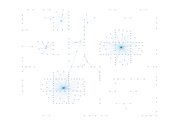### Degree distribution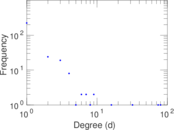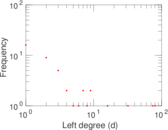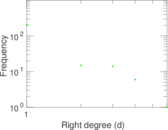### Cumulative degree distribution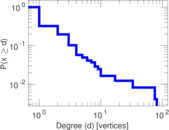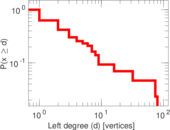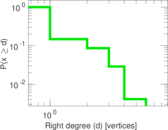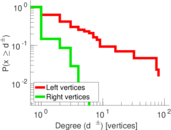### Lorenz curve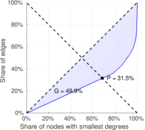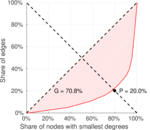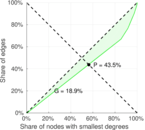### Spectral distribution of the adjacency matrix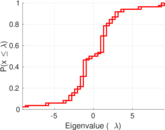### Spectral distribution of the normalized adjacency matrix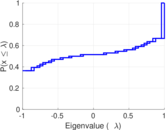### Spectral distribution of the Laplacian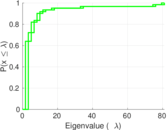### Spectral graph drawing based on the adjacency matrix### Spectral graph drawing based on the Laplacian### Spectral graph drawing based on the normalized adjacency matrix### Degree assortativity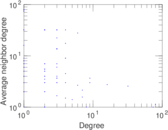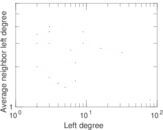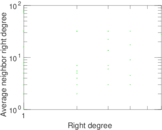### Zipf plot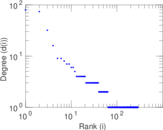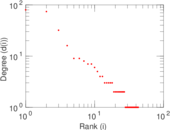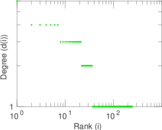### Hop distribution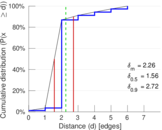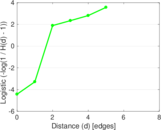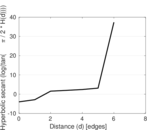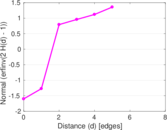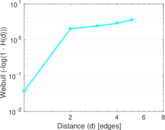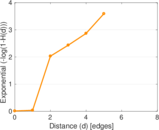### Double Laplacian graph drawing### Delaunay graph drawing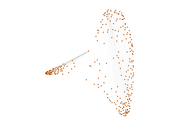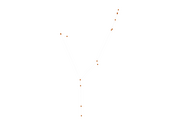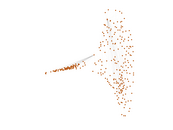### Edge weight/multiplicity distribution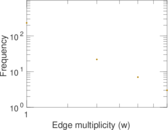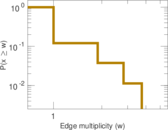### Temporal distribution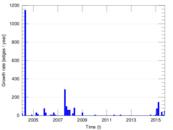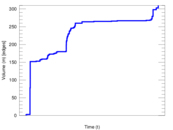### Temporal hop distribution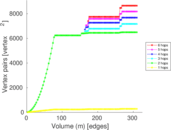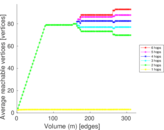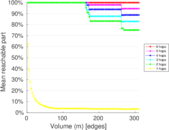### Diameter/density evolution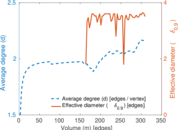### Matrix decompositions plots